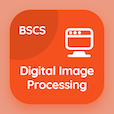Computer Science Courses

Database Systems Practice Tests

Database Systems Tests

The Book Domain Relational Calculus Multiple Choice Questions (MCQ Quiz) PDF, Domain Relational Calculus MCQs, download to learn online database systems courses. Study Formal Relational Query Languages Multiple Choice Questions and Answers (MCQs), Domain Relational Calculus quiz answers PDF for online college classes. The eBook Domain Relational Calculus MCQ App Download: relational algebra, domain relational calculus test prep for applied computer science.

The MCQ: A second form of relational calculus, is known as PDF, "Domain Relational Calculus MCQ" App Download (Free) with domain relational calculus, relational calculus, tuple relational calculus, and attribute relational calculus choices for online college classes. Practice domain relational calculus quiz questions, download Google eBook (Free Sample) for accelerated computer science degree online.

## Database Systems: Domain Relational Calculus MCQs

MCQ: A second form of relational calculus, is known as

A) Domain relational calculus
B) Relational calculus
C) Tuple relational calculus
D) Attribute relational calculus

MCQ: The restricted tuple relational calculus is equivalent to the

A) Tuple relational calculus
B) Attribute relational calculus
C) Domain relational calculus
D) Relational algebra

MCQ: In domain relational calculus, all values that appear in tuples of the expression are values from

A) Views
B) Domain
C) Relation
D) Aggregation

### Practice Tests: Database Systems Exam Prep

Download Database Management System Quiz App, Computer Networks MCQ App, and Digital Image Processing MCQs App to install for Android & iOS devices. These Apps include complete analytics of real time attempts with interactive assessments. Download Play Store & App Store Apps & Enjoy 100% functionality with subscriptions!